# What Is A Skewed Distribution? (5 Key Things You Should Know)

Many of the distributions we work with in real life will be somewhat skewed, without the symmetry that we see in a normal distribution.

So, what is a skewed distribution?  A skewed distribution is asymmetric, meaning it has a long “tail”, and there is no value that gives us a mirror image. Skewness is a number that measures the asymmetry of a skewed distribution. A symmetric distribution has zero skewness, but zero skewness does not imply a symmetric distribution.

Of course, a skewed distribution can be both positively skewed (right-skewed) and negatively skewed (left-skewed).

In this article, we’ll talk about what a skewed distribution is and what it looks like.  We will also answer some common questions about skewed distributions.

Let’s get started.

## What Is A Skewed Distribution?

A skewed distribution is an asymmetric probability distribution.  By asymmetric, we mean that there are more data points (or more probability, or more weight) on one side of the mean than the other (as illustrated in the picture below).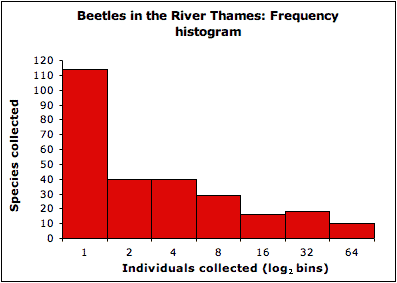This is a skewed distribution. There is more data on the left side, and there is a long tail on the right side, meaning the distribution is right-skewed (or positively skewed).

Here is another way to think of a skewed distribution: if we tried to balance the middle of the distribution on a thin fulcrum, it would tip over or tilt to one side, due to the uneven weight.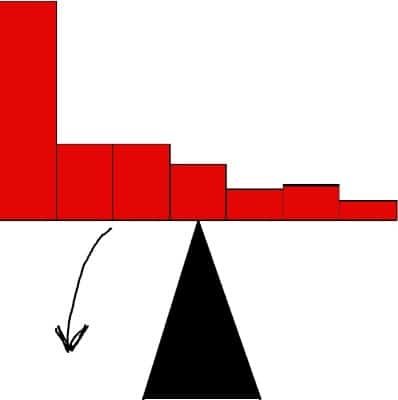If your distribution will not balance on a fulcrum, then it is skewed.

As you can see in the picture, there is more data on one side of the mean (left) than there is on the other side (right). Another way to say this is that there is more probability (or more area under the curve) on one side than there is on the other side.

5 Common Misunderstandings About Ph...
5 Common Misunderstandings About Physics

Skewness is a numerical measure of the asymmetry of a skewed distribution.  We can measure skew for both unimodal (one mode) and multimodal (more than one mode) data sets.

A symmetric distribution has the same mean and median, and it also has zero skewness.  However, zero skewness does not always mean that a distribution is symmetric.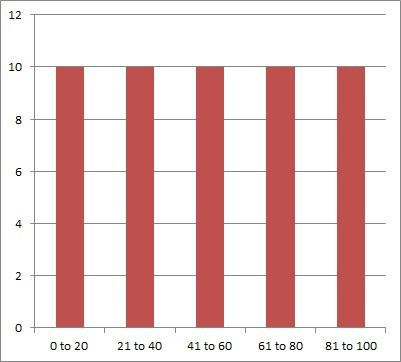A symmetric distribution (like this uniform distribution) has the same mean and median. It also zero skewness due to its symmetry.

A symmetric unimodal distribution has the same mean, median, and mode, and it also has zero skewness.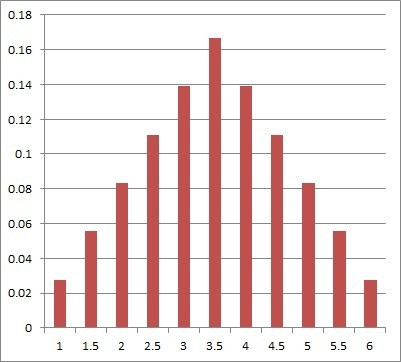A symmetric unimodal distribution like this one has the same mean, median, and mode. It also has zero skewness due to its symmetry.

There are two basic types of skewed distributions: positively skewed (right-skewed) and negatively skewed (left-skewed).  The words right and left tell you which direction the long tail of the distribution is pointing.

### What Is A Positively Skewed Distribution (Right-Skewed Distribution)?

A positively skewed distribution (right-skewed distribution) is one where the right tail (the part closer to positive values) is longer.  In this case, there are more data values (or more probability) to the left of the mean than to the right of the mean.

A positively skewed distribution looks like it is leaning to the left, as you can see in the picture below.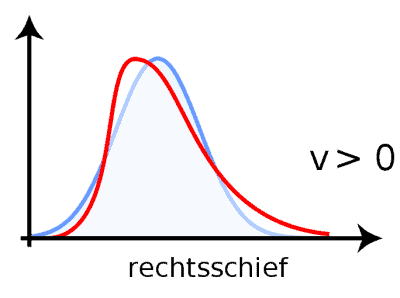A positively skewed (or right-skewed) distribution (red curve) looks like it is leaning to the left. The blue curve is a symmetric unimodal distribution (with zero skewness) for comparison.

The blue and red curves in the picture above have the same area under the curve (1 for a probability distribution). However, the skewness varies (symmetric for the blue curve, right-skewed for the red curve).

### What Is A Negatively Skewed Distribution (Left-Skewed Distribution)?

A negatively skewed distribution (left-skewed distribution) is one where the left tail (the part closer to negative values) is longer.  In this case, there are more data values (or more probability) to the right of the mean than to the left of the mean.

A negatively skewed distribution looks like it is leaning to the right, as you can see in the picture below.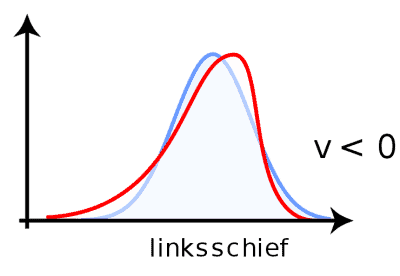A negatively skewed (or left-skewed) distribution (red curve) looks like it is leaning to the right. The blue curve is a symmetric unimodal distribution (with zero skewness) for comparison.

The blue and red curves in the picture above have the same area under the curve (1 for a probability distribution). However, the skewness varies (symmetric for the blue curve, left-skewed for the red curve).

### What Can Cause A Skewed Distribution?

One or more extreme data values can cause a skewed distribution. The extreme values can occur on one side or the other, and this will tell us which way the distribution is skewed.

For example, a few very high salaries and many average salaries at a company will result in a distribution that is skewed to the right.A few high salaries at a company, along with many average salaries, can cause a salary distribution that is skewed to the right (more employees on the left with average salaries, with a few way out on the right with high salaries).

Similarly, a few very thin people and many average weight people will result in a distribution that is skewed to the left.A few very thin people in a group, along with many average weight people, can cause a weight distribution that is skewed to the left (more people on the right with average weights, with a few way out on the left with low weights).

### Does A Skewed Distribution Have A Standard Deviation?

A skewed distribution has a standard deviation, just like any other distribution. We can calculate it with the usual formula (the formula for a discrete distribution is shown below), which requires us to find the mean of the distribution first.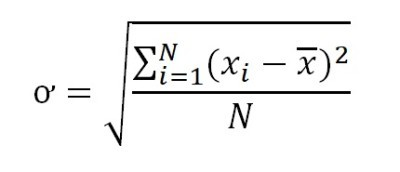This is the formula to calculate the standard deviation for a discrete probability distribution.

However, the standard deviation that we calculated may not tell us much about the distribution in terms of skewness (symmetry).

For example, a normal distribution can have a standard deviation of 1, 10, or 100 depending on the data, but it will always be symmetric (with zero skewness). You can see this in the picture below.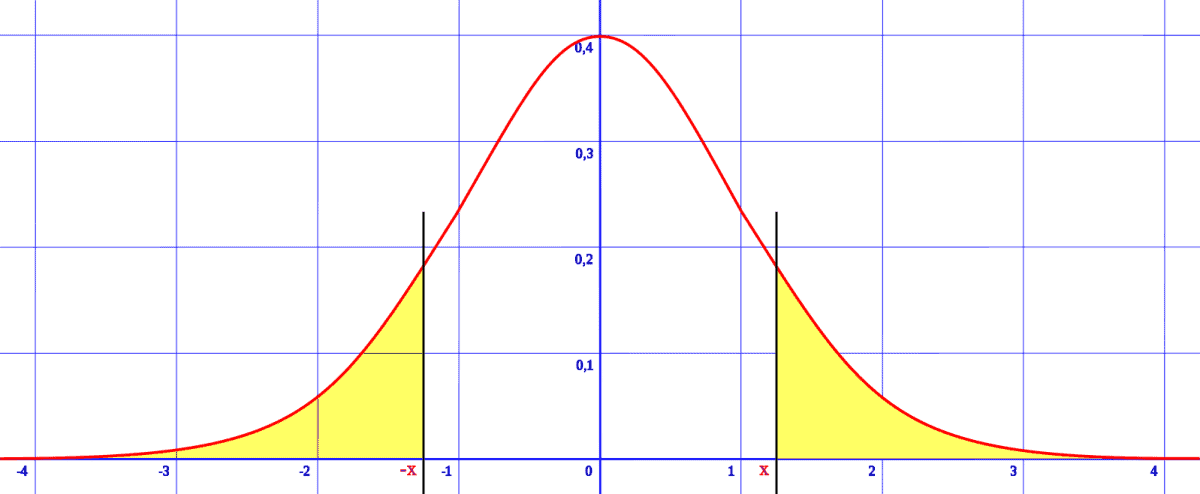A normal distribution can have a standard deviation of 1, 10, 100, etc., but it will always be symmetric about the mean, with zero skewness.

On the other hand, the standard deviation for a bimodal distribution could have the same values as mentioned above (1, 10, or 100), but it may not be symmetric.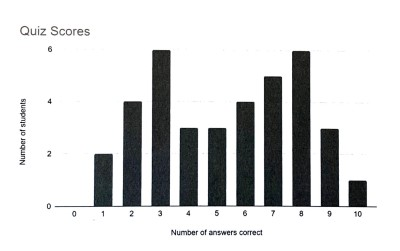A bimodal distribution has two modes, and we can calculate a standard deviation. However, this particular bimodal distribution is not symmetric.

If a bimodal distribution is symmetric, then the mean will be the average of the two modes (halfway in between the two modes). You can see an example of a symmetric bimodal distribution in the picture below.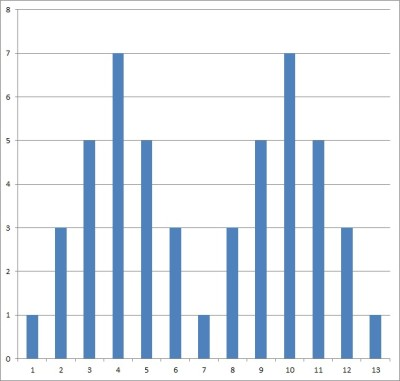This is a symmetric bimodal distribution with zero skewness (there is no long tail on either side).

### Can A Skewed Distribution Also Be Symmetric?

A skewed distribution cannot also be symmetric, since these two concepts are mutually exclusive.

If a distribution is skewed, then it is not symmetric.  As a result, we can also say that a skewed distribution cannot be uniform or normal.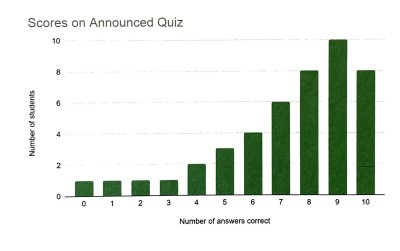This distribution is skewed (in particular, left-skewed or negatively skewed). As a result, it is not symmetric.

If a distribution is symmetric, then it is not skewed (since the skewness is zero).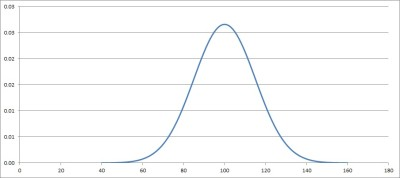This normal distribution is symmetric. As a result, it is not skewed (and it has a skewness of zero).

### Can You Standardize A Skewed Distribution?

You can standardize a skewed distribution, just like you can standardize any distribution.  The way to standardize is to subtract the mean M and divide by the standard deviation S of the original distribution.

The transformation is a linear function given by f(X) = (X – M) / S. We can also express this in the form Y = AX + B, where A = 1/S and B = -M/S.

This gives us a new variable, Y = (X – M) / S, which has a mean of 0 and a standard deviation of 1.

However, this new distribution will not be normal if the original distribution was not normal.  Standardizing a distribution changes the values of each individual data point, but it does not change the general shape of the distribution.

In other words, a linear transformation f(X) = AX + B cannot add or remove skew from a distribution.

### Can A Bimodal Distribution Be Skewed?

A bimodal distribution can be skewed or symmetric, depending on the situation. However, it cannot be both skewed and symmetric, as we mentioned earlier.

For example, the bimodal distribution below is symmetric, with a skewness of zero.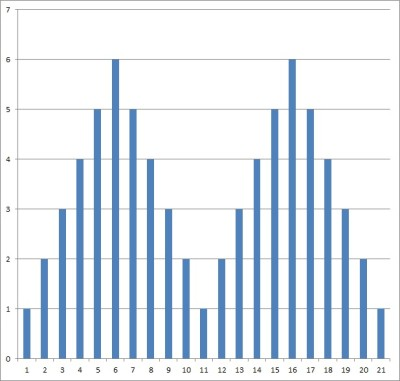This bimodal distribution is symmetric, with a skewness of zero.

However, the bimodal distribution below is asymmetric and right skewed.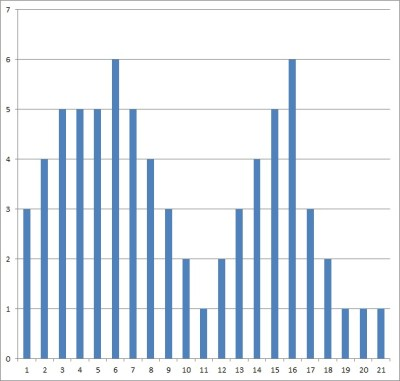This bimodal distribution is asymmetric, and it is right-skewed (positively skewed) with a long tail on the right.

### Can A Normal Distribution Be Skewed?

A normal distribution cannot be skewed.  Remember that a normal distribution is symmetric about the mean: the left and right halves are mirror images.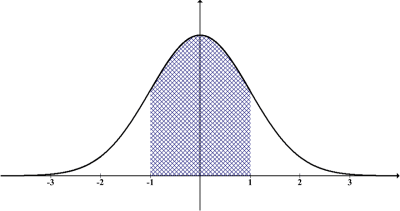A normal distribution is symmetric, so it cannot be skewed.

Note that a symmetric distribution has zero skewness, so a normal distribution has zero skewness.

## Conclusion

Now you know what skewed distributions are and what they can look like.  You also know the answers to some common questions about skewed distributions.

The sampling distribution for a skewed distribution can still be normal for a large enough sample size – you can learn more here.

I hope you found this article helpful.  If so, please share it with someone who can use the information.

~Jonathon